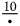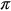# namjaaaa

Lv5

## Cagayan State University

11 Followers
2 Following
1 Helped

Published53

### Subjects

English13Marketing2Algebra2Mathematics34Chemistry2
Answer: Exact Form: 5/4 Decimal Form: 1.25
Answer: Take the derivative of the numerator and denominator, then evaluate th...
Answer: Vertical Asymptotes: x=2x=2 Horizontal Asymptotes: y=2y=2 No Oblique A...
Answer: Vertical Asymptotes: x=2x=2 Horizontal Asymptotes: y=2y=2 No Oblique A...
Answer:O -2 O 1 O 2.
Answer HELLO CAN YOU SEND IT HERE. THANK YOU
Answer: 4 6 8 10 12 14 16 18 58387 54159770
Answer: Planning dinner parties How about that inevitable dinner party or cock...
Answer:Mathematical concepts based on cultural perspectives allow students to ...
Answer: = circumference = the constant pi = radius of the circle
Answer: = circumference = the constant pi = radius of the circle
Answer: A semicircle is a closed 2D shape. It is not a polygon since it has a ...
Answer: A semicircle is a closed 2D shape. It is not a polygon since it has a ...
Answer: i feel stress. Yes, because Mathematics makes our life orderly and pre...
1.) f[g(x)]
Part 2. Fractions take input, operate on the input, and generate an output. Sometimes the Input can bea nother function. The output represents the composition of functions. Try it!
f(x)=2 x+3              h[x] = x-2            f (x) =a) f[h()]
b) f[h(x)]
c) h[j()]
d.) h[j(x)]

Phew all of these parenthesis are getting tiring to write. When problems like (f) are read aloud it would sound like "h of j of x ". Mathematicians saw this double "of" and replaced with this symbol * . When you see it think "of". A problem like h[j(x)] could also be written as h = j and would be read "h of j". Try it!

g)   h[(7)]
h.)   h [f()]
i.)    h[f(x)] = hf
j.)    fh
k.)f
l.)    hh
m.)  ff
n.)   j[h(2)]

Answer: pls picture it clearly. Thank you
Answer: 1. Yes, the children are born ready. 2. the skills would be: strength ...